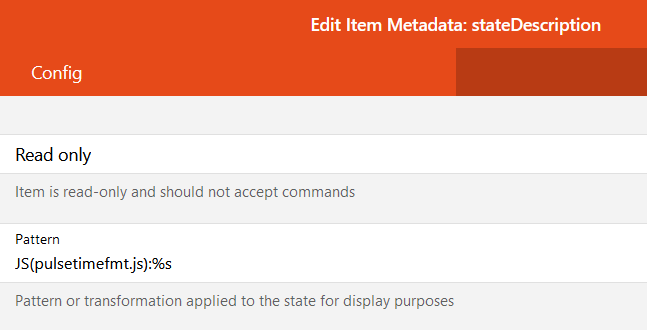# Pulsetime display

I use some Sonoff devices flashed with tasmota. I set pulsetime with rules but would like to display them in a user friendly way,

For example, pulsetime 700 = 10 min (calculation is (700-100)/60)). Is this possible specifying a “State Description” metadata in the item ? How ?

1 Like

This thread may help with conversion secs->mins for display, using a JS transformation in the [state presentation]

EDIT - seem to have missed the link, sorry

1 Like

@rossko57 Thanks. Based of your post here is the solution:

1. Install Javascript transformation
2. Create `pulsetimefmt.js` in the `transform` folder with this content
``````/*
Javascript transform function to format a Pulsetime duration
into a more readable clock-like format
*/

(function(i) {
var dur = i;
var stringHours = '';
var stringMinutes = '';
var stringSeconds = '';
if (dur == 0) { // pulsetime is OFF
stringSeconds = 'OFF';
}
if (dur <= 111) { // small pulsetime
dur = dur/10;
}
if (dur >= 112) { // large pulsetimeF
dur = dur-100;
}
var hours = 0;
var minutes = 0;
var seconds = dur;
if (dur >= 60) { // 60 seconds in a minute
minutes = Math.floor(dur / 60); // Number of minutes
seconds = dur - (minutes * 60); // Remove minutes from seconds bucket
}
if (minutes >= 60) { // 60 minutes in an hour
hours = Math.floor(minutes / 60); // Number of hours
minutes = minutes - (hours * 60); // Remove hours from minutes bucket
}
// Let's format
if (hours > 0) { stringHours = hours + 'h ' }
if (minutes > 0) { stringMinutes = minutes + 'm ' }
if (seconds > 0) { stringSeconds = seconds + 's' }
// now build the output
var returnString =  stringHours + stringMinutes + stringSeconds
return returnString.trim(); // Removes trailing spaces

})(input)
``````
1. Parametrize Metadata -> State Description as follows